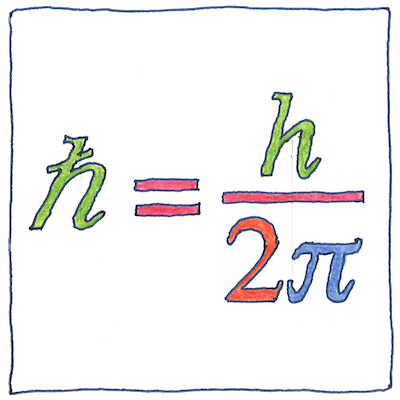1900

The book of science

Tom Sharp

 Max Planck quantum mechanics

Planck constant

• The energy of a phonon
• is related to its frequency
• by the Planck constant, h,
• as E = hf.
• Max Planck calculated h
• to explain the nature
• of black-body
• but in 1900
• Planck realized that h
• was the smallest unit
• of action
• the basis of everything,
• not just photons,
• but all energy
• and all matter.

Planck units

• A natural unit of measurement
• is based on a property of nature.
• A property of nature like
• the least largest unit of motion.
• The dimensions of motion
• are energy and time,
• and its value can be expressed
• in joule-seconds, or in Plancks.
• Fundamental units
• for mass, length, and time,
• therefore, for all physical quantities,
• can be expressed in terms of
• ℏ, the reduced Planck’s constant,
• G0, Newton’s gravitational constant,
• ke, Coulomb’s constant for electric force,
• kB, Boltzmann’s constant for kinetic energy,
• and c, the velocity of light.
• These, with clever arrangements
• of powers and roots,
• give us natural units for
• Planck length,
• Planck mass,
• Planck time,
• Planck charge,
• and Planck temperature.

Gyroscope

• Even an imperfect gyroscope
• maintains its vertical to conserve
• angular momentum.
• If you could harness a perfect gyroscope,
• it could turn the hand of a clock
• once every day,
• but the harness would impair
• the gyroscope so that it would thus
• be imperfect.

The Planck constant led directly to the discovery that nothing in the physical universe is strictly continuous; instead, everything is quantized. The Planck constant reduced by dividing by 2π (ℏ or h-bar) is the quantum of angular momentum (the smallest possible piece of orbital or rotating energy) and helps determine the sizes of the Bohr magneton and other neat little units of our universe.

See also in The book of science: